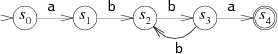# Regexp tutorial and cheat sheet

yourbasic.org/golang

A regular expression is a sequence of characters that define a search pattern.## Basics

The regular expression `a.b` matches any string that starts with an `a`, ends with a `b`, and has a single character in between (the period matches any character).

To check if there is a substring matching `a.b`, use the regexp.MatchString function.

``````matched, err := regexp.MatchString(`a.b`, "aaxbb")
fmt.Println(matched) // true
fmt.Println(err)     // nil (regexp is valid)``````

To check if a full string matches `a.b`, anchor the start and the end of the regexp:

• the caret `^` matches the beginning of a text or line,
• the dollar sign `\$` matches the end of a text.
``````matched, _ := regexp.MatchString(`^a.b\$`, "aaxbb")
fmt.Println(matched) // false``````

Similarly, we can check if a string starts with or ends with a pattern by using only the start or end anchor.

### Compile

For more complicated queries, you should compile a regular expression to create a `Regexp` object. There are two options:

``````re1, err := regexp.Compile(`regexp`) // error if regexp invalid
re2 := regexp.MustCompile(`regexp`)  // panic if regexp invalid``````

### Raw strings

It’s convenient to use ``raw strings`` when writing regular expressions, since both ordinary string literals and regular expressions use backslashes for special characters.

A raw string, delimited by backticks, is interpreted literally and backslashes have no special meaning.

## Cheat sheet

### Choice and grouping

Regexp Meaning
`xy` `x` followed by `y`
`x|y` `x` or `y`, prefer `x`
`xy|z` same as `(xy)|z`
`xy*` same as `x(y*)`

### Repetition (greedy and non-greedy)

Regexp Meaning
`x*` zero or more x, prefer more
`x*?` prefer fewer (non-greedy)
`x+` one or more x, prefer more
`x+?` prefer fewer (non-greedy)
`x?` zero or one x, prefer one
`x??` prefer zero
`x{n}` exactly n x

### Character classes

Expression Meaning
`.` any character
`[ab]` the character a or b
`[^ab]` any character except a or b
`[a-z]` any character from a to z
`[a-z0-9]` any character from a to z or 0 to 9
`\d` a digit: `[0-9]`
`\D` a non-digit: `[^0-9]`
`\s` a whitespace character: `[\t\n\f\r ]`
`\S` a non-whitespace character: `[^\t\n\f\r ]`
`\w` a word character: `[0-9A-Za-z_]`
`\W` a non-word character: `[^0-9A-Za-z_]`
`\p{Greek}` Unicode character class*
`\pN` one-letter name
`\P{Greek}` negated Unicode character class*
`\PN` one-letter name

### Special characters

To match a special character `\^\$.|?*+-[]{}()` literally, escape it with a backslash. For example `\{` matches an opening brace symbol.

Other escape sequences are:

Symbol Meaning
`\t` horizontal tab = `\011`
`\n` newline = `\012`
`\f` form feed = `\014`
`\r` carriage return = `\015`
`\v` vertical tab = `\013`
`\123` octal character code (up to three digits)
`\x7F` hex character code (exactly two digits)

### Text boundary anchors

Symbol Matches
`\A` at beginning of text
`^` at beginning of text or line
`\$` at end of text
`\z`
`\b` at ASCII word boundary
`\B` not at ASCII word boundary

### Case-insensitive and multiline matches

To change the default matching behavior, you can add a set of flags to the beginning of a regular expression.

For example, the prefix `"(?is)"` makes the matching case-insensitive and lets `.` match `\n`. (The default matching is case-sensitive and `.` doesn’t match `\n`.)

Flag Meaning
`i` case-insensitive
`m` let `^` and `\$` match begin/end line in addition to begin/end text (multi-line mode)
`s` let `.` match `\n` (single-line mode)

## Code examples

### First match

Use the `FindString` method to find the text of the first match. If there is no match, the return value is an empty string.

``````re := regexp.MustCompile(`foo.?`)
fmt.Printf("%q\n", re.FindString("seafood fool")) // "food"
fmt.Printf("%q\n", re.FindString("meat"))         // ""``````

### Location

Use the `FindStringIndex` method to find `loc`, the location of the first match, in a string `s`. The match is at `s[loc:loc]`. A return value of nil indicates no match.

``````re := regexp.MustCompile(`ab?`)
fmt.Println(re.FindStringIndex("tablett"))    // [1 3]
fmt.Println(re.FindStringIndex("foo") == nil) // true``````

### All matches

Use the `FindAllString` method to find the text of all matches. A return value of nil indicates no match.

The method takes an integer argument `n`; if `n >= 0`, the function returns at most `n` matches.

``````re := regexp.MustCompile(`a.`)
fmt.Printf("%q\n", re.FindAllString("paranormal", -1)) // ["ar" "an" "al"]
fmt.Printf("%q\n", re.FindAllString("paranormal", 2))  // ["ar" "an"]
fmt.Printf("%q\n", re.FindAllString("graal", -1))      // ["aa"]
fmt.Printf("%q\n", re.FindAllString("none", -1))       // [] (nil slice)``````

### Replace

Use the `ReplaceAllString` method to replace the text of all matches. It returns a copy, replacing all matches of the regexp with a replacement string.

``````re := regexp.MustCompile(`ab*`)
fmt.Printf("%q\n", re.ReplaceAllString("-a-abb-", "T")) // "-T-T-"``````

### Split

Use the `Split` method to slice a string into substrings separated by the regexp. It returns a slice of the substrings between those expression matches. A return value of nil indicates no match.

The method takes an integer argument `n`; if `n >= 0`, the function returns at most `n` matches.

``````a := regexp.MustCompile(`a`)
fmt.Printf("%q\n", a.Split("banana", -1)) // ["b" "n" "n" ""]
fmt.Printf("%q\n", a.Split("banana", 0))  // [] (nil slice)
fmt.Printf("%q\n", a.Split("banana", 1))  // ["banana"]
fmt.Printf("%q\n", a.Split("banana", 2))  // ["b" "nana"]

zp := regexp.MustCompile(`z+`)
fmt.Printf("%q\n", zp.Split("pizza", -1)) // ["pi" "a"]
fmt.Printf("%q\n", zp.Split("pizza", 0))  // [] (nil slice)
fmt.Printf("%q\n", zp.Split("pizza", 1))  // ["pizza"]
fmt.Printf("%q\n", zp.Split("pizza", 2))  // ["pi" "a"]``````

#### More functions

There are 16 functions following the naming pattern

`Find(All)?(String)?(Submatch)?(Index)?`

For example: `Find`, `FindAllString`, `FindStringIndex`, …

• If `All` is present, the function matches successive non-overlapping matches.
• `String` indicates that the argument is a string; otherwise it’s a byte slice.
• If `Submatch` is present, the return value is a slice of successive submatches. Submatches are matches of parenthesized subexpressions within the regular expression. See `FindSubmatch` for an example.
• If `Index` is present, matches and submatches are identified by byte index pairs.

## Implementation

• The `regexp` package implements regular expressions with RE2 syntax.
• It supports UTF-8 encoded strings and Unicode character classes.
• The implementation is very efficient: the running time is linear in the size of the input.
• Backreferences are not supported since they cannot be efficiently implemented.

### Further reading